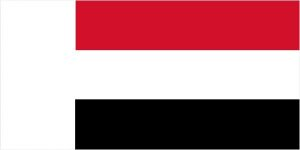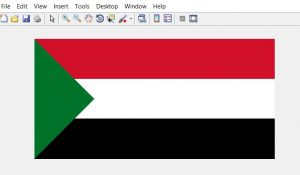Related Articles

# Draw Sudan Flag using MATLAB

• Last Updated : 14 Mar, 2019

In Digital image processing, a colored image is represented in a 3-Dimensional matrix. Image can be represented in various color models such as RGB (Red, Green, Blue) model, HSV (Hue, Saturation, Value) model, YIQ (Luminance-Inphase Quadrature) model, CMYK (Cyan, Magenta, Yellow, Black) model. Generally, an image is represented in the RGB model. The first channel of the matrix is Red, the second channel is the Green and the third channel is Blue.

Approach to draw Sudan Flag:

• Create a zero matrix of 300X600X3.300 is the number of rows, 600 is the number of columns and 3 represent RGB color channel. Fill the matrix with (255, 255, 255). 255 represent white pixel and 0 represent black pixel.
`img(:, :, :)=255`
• Fill the matrix with crimson color(210, 16, 42) between rows from 1 to 100 and between columns from 151:600.
• Fill the matrix with black(0, 0, 0) between rows from 200 to 300 and between columns from 151:600.
• After these operations, our image looks like this• Now, we have to draw one upper triangle and one lower triangle.
• For lower triangle, from row 1 to 150 and column 1 to 150 fill the matrix with green color(0, 114, 14)
• Similarly, for upper triangle, from row 150 to 300 and column 1 to 150 fill the matrix with green color(0, 114, 14)

Implementation is given below:

 `% MATLAB code to draw Sudan flag`` ` `% initialising a zero matrix of 300X600X3``img=uint8(zeros(300, 600, 3));``img(:, :, :)=255;``%Red Color``img(1:100, 151:600, 1)=210;``img(1:100, 151:600, 2)=16;``img(1:100, 151:600, 3)=42;``%black Color ``img(200:300, 150:600, :)=0;``% Lower Triangle``for` `i=1:150``    ``for` `j=1:150``        ``if``(j=200)``            ``img(i, j, :)=0;            ``        ``end``    ``end``end`` ` `% displaying the matrix as image``figure, imshow(img);`

Output :Attention geek! Strengthen your foundations with the Python Programming Foundation Course and learn the basics.

To begin with, your interview preparations Enhance your Data Structures concepts with the Python DS Course. And to begin with your Machine Learning Journey, join the Machine Learning – Basic Level Course

My Personal Notes arrow_drop_up